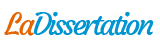# ROCE Carrefour

Étude de cas : ROCE Carrefour. Recherche parmi 289 000+ dissertations

Par   •  17 Février 2023  •  Étude de cas  •  302 Mots (2 Pages)  •  84 Vues

Page 1 sur 2

FINACIAL MANAGEMENT EXPOSÉ

Now we will calculate Carrefour’s ROCE for the last two years. But first, the Return on Capital Employed is a financial ratio that can be used to assess a company’s profitability and capital efficiency. The ROCE percentage can be particularly useful for comparing the performance of capital-intensive enterprises.

The ROCE is calculated by:

Earnings Before Interest and Taxes or EBIT

(/)

Average Capital Employed

=

Return on Capital Employed

In more detail, these are:

ROCE = BAII N / ((Employee Capital N-1 + Employee Capital N) / count)

For 2021, we have:

ROCE = BAII 2021 / ((Employee Capital 2020 + Employee Capital 2021) / 2)

• BAII also known as EBIT includes all expenses except interest and income taxes. That’s the difference between operating revenues and operating expenses. The 2021 EBIT is 1927.

• Capital employed 2020 is calculated as Total Assets - Total Current Liabilities. We have 47,588 - 21,342 = 26 246

• Capital employed 2021 is calculated by also Total Assets - Total Current Liabilities, which gives us 47668 – 22150 = 25 518.

Finally, we have,

ROCE =        1927        / ((26246        +        25518)        / 2)

ROCE =        1927        /        25882

ROCE =        7,45 %

Pour 2020,

ROCE = BAII 2022 / ( (Capital Employé 2021 + Capital employé 2022) / 2 )

ROCE = 1556 / ( ((47668 - 22150) + (52755 - 24417)) / 2 )

ROCE = 1556 / ( ( 25518 + 28338 ) / 2 )

ROCE = 1556 / 26928

ROCE = 5,78 %

...

Télécharger au format  txt (1.5 Kb)   pdf (40.1 Kb)   docx (197.5 Kb)
Voir 1 page de plus »